# Hypervolume-Resampling

library(hypervolume)
library(palmerpenguins)
library(ggplot2)
library(gridExtra)
set.seed(123)
data(penguins)
data(quercus)

# Introduction to Resampling Hypervolumes

When working with the package hypervolume, it is important to understand the statistical significance of the resulting hypervolume or hypervolumes. The methods introduced in this update are meant to characterize both variance from data sampling and variance due to non-deterministic behavior in the hypervolume algorithms.

This update to the package provides the following functionalities:
- an interface for generating large resamples of hypervolumes
- methods for generating non-parametric confidence intervals for hypervolume parameters and null distributions for overlap statistics
- formal statistical tests based on hypervolumes

The purpose of this document is to provide use cases and explain best practices when using the new methods. The examples are chosen to highlight all the considerations that go into interpreting results.

## Use case 1: Effect of sample size on volume

The following code demonstrates visualizing the effect of sample size on hypervolumes constructed using Gaussian kernels. Thirty hypervolumes are constructed per sample size.

To plot how a summary statistic describing a hypervolume varies with sample size, a function must be passed to the func field of hypervolume_funnel. A user inputted function must take a hypervolume object as an input and output a numeric. By default, func = get_volume. The confidence intervals in the plots are generated non-parametrically by taking quantiles at each sample size. When using hypervolume_funnel to plot the output of hypervolume_resample, a ggplot object is returned. It is then possible to add more plot elements to the result.

# Run time with cores = 2 is around 25 minutes
hv = hypervolume(na.omit(penguins)[,3:4], verbose = FALSE)
resample_seq_path = hypervolume_resample("penguins_hvs", hv, method = "bootstrap seq", n = 30, seq = c(100, 125, 150, 175, 200, 225, 250, 275, 300), cores = 20)

hypervolume_funnel(resample_seq_path, title = "Volume of Hypervolumes at Different Resample Sizes") + ylab("Volume")

plot1 = hypervolume_funnel(resample_seq_path, title = "Mean of Bill Length at Different Resample Sizes",
func = function(x) {get_centroid(x)["bill_length_mm"]}) +
ylab("Bill Length (mm)")

plot2 = hypervolume_funnel(resample_seq_path, title = "Mean of Bill Depth at Different Resample Sizes",
func = function(x) {get_centroid(x)["bill_depth_mm"]}) +
ylab("Bill Depth (mm)")

grid.arrange(plot1, plot2, nrow = 2)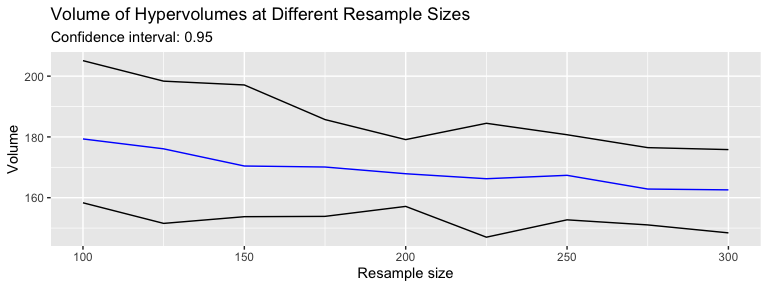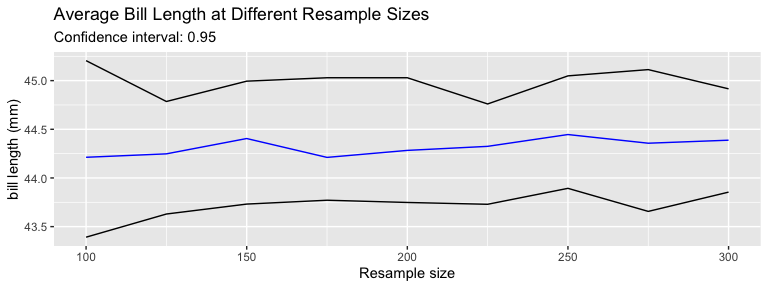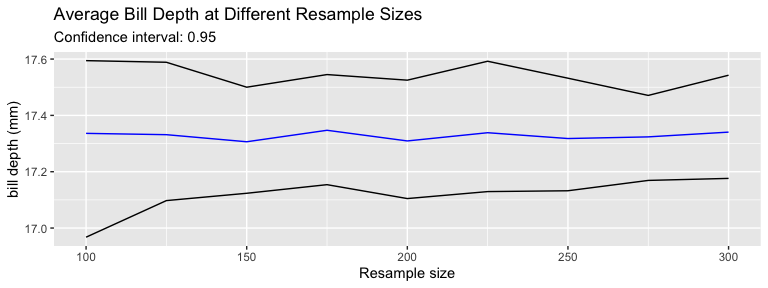The default contruction of hypervolumes uses kde.bandwidth = estimate_bandwidth(data, method = "silverman"). The first plot shows that volume decreases with sample size due to Silverman bandwidth decreasing with sample size. In fact, Silverman bandwidth is not appropriate for multimodal data such as penguins. The plot demonstrates this fact and shows that at small sample size, the hypervolume overestimates the true volume. Other methods for estimating bandwidth may be more accurate, but are computationally unfeasible for data with more than 3 dimensions. The estimated volume converges to the true volume of the population as sample size increases; however, at 300 data points, the result from hypervolume_funnel suggests that the volume is being overestimated.

In contrast, the plots for the mean of each column of data show that the centroid of the data is preserved by hypervolume construction using gaussian kernels.

In the example, each confidence interval is a quantile of 30 resampled values. Improving the accuracy requires larger sample sizes which drastically increases run time. It is recommended to use more cores to allow hypervolumes to be generated in parallel; however, by default, cores = 1 and the function runs sequentially.

## Use case 2: Effect of simulating bias when resampling

The following code demonstrates the effect of applying a bias while resampling data. In the example, we use penguins data to construct a hypervolume object, then resample it while biasing towards large beak sizes. In this example, this is done by more strongly weighting the points closer to the maximum observed values when resampling.

Weights can be applied when resampling points by either passing a user defined function to the weight_func field of hypervolume_resample, or by specifying the mu and sigma parameters. When using mu and sigma, the weight function is a multivariate normal distribution. mu is the mean of multivariate normal distribution while sigma is the diagonal covariance matrix of a multivariate normal distribution. cols_to_bias specifies which columns to use as the input of the weight function.

hv = hypervolume(na.omit(penguins)[,3:6], verbose = FALSE)
#> Warning in hypervolume(na.omit(penguins)[, 3:6], verbose = FALSE):
#> Consider removing some axes.
#> Warning in hypervolume(na.omit(penguins)[, 3:6], verbose = FALSE): Some dimensions have much more higher standard deviations than others:
#>  bill_length_mm 5.47
#>  bill_depth_mm 1.97
#>  flipper_length_mm 14.02
#>  body_mass_g 805.22
#> Consider rescaling axes before analysis.
#> Note that the formula used for the Silverman estimator differs in version 3 compared to prior versions of this package.
#> Use method='silverman-1d' to replicate prior behavior.
cols_to_bias = c("bill_length_mm", "bill_depth_mm")
mu = apply(hv@Data, 2, max)[cols_to_bias]
sigma = apply(hv@Data, 2, var)[cols_to_bias]*2
biased_path = hypervolume_resample("Bill bias", hv, method = "biased bootstrap", n = 1, mu = mu, sigma = sigma, cols_to_bias = cols_to_bias)
#> Warning: executing %dopar% sequentially: no parallel backend registered
#> Note that the formula used for the Silverman estimator differs in version 3 compared to prior versions of this package.
#> Use method='silverman-1d' to replicate prior behavior.

# Read in hypervolume object from file
biased_hv = readRDS(file.path(biased_path, "resample 1.rds"))

combined_dat = data.frame(rbind(hv@Data, biased_hv@Data))
combined_dat['Type'] = rep(c('original', 'biased'), each = nrow(hv@Data))
plot1 = ggplot(combined_dat, aes(y = ..density..)) + geom_histogram(aes(x = bill_depth_mm, fill = Type), bins = 20) +
facet_wrap(~Type) +
ggtitle("Distribution of Bill Depth", "Biased resample vs Original sample") +
xlab("bill depth (mm)")
plot2 = ggplot(combined_dat, aes(y = ..density..)) + geom_histogram(aes(x = bill_length_mm, fill = Type), bins = 20) +
facet_wrap(~Type) +
ggtitle("Distribution of Bill Length", "Biased resample vs Original sample") +
xlab("bill length(mm)")
grid.arrange(plot1, plot2, nrow = 2)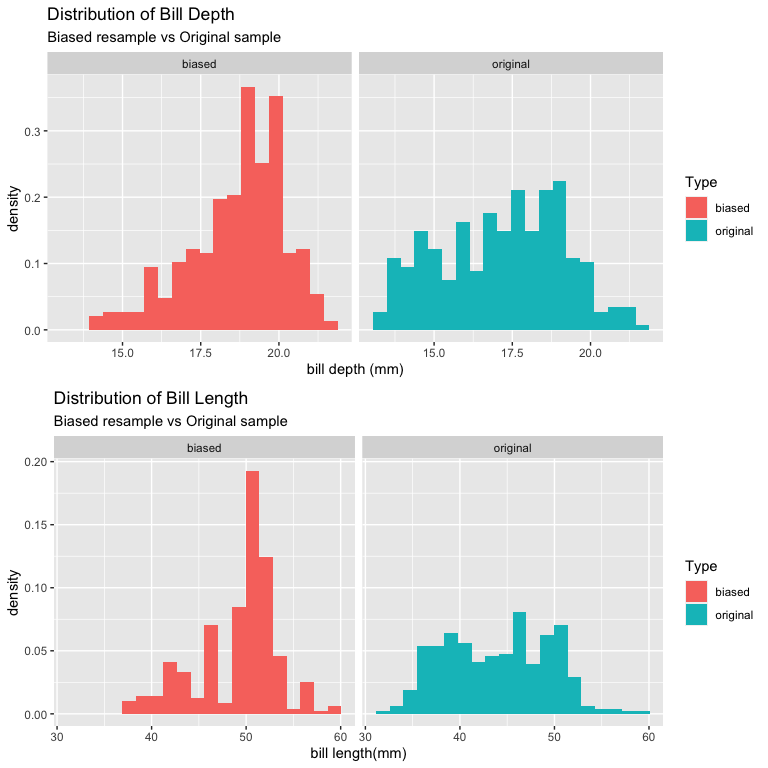plot1 = ggplot(combined_dat, aes(y = ..density..)) + geom_histogram(aes(x = flipper_length_mm, fill = Type), bins = 20) +
facet_wrap(~Type) +
ggtitle("Distribution of Flipper Length", "Biased resample vs Original sample") +
xlab("flipper length (mm)")
plot2 = ggplot(combined_dat, aes(y = ..density..)) + geom_histogram(aes(x = body_mass_g, fill = Type), bins = 20) +
facet_wrap(~Type) +
ggtitle("Distribution of Body Mass", "Biased resample vs Original sample") +
xlab("body mass (g)")
grid.arrange(plot1, plot2, nrow = 2)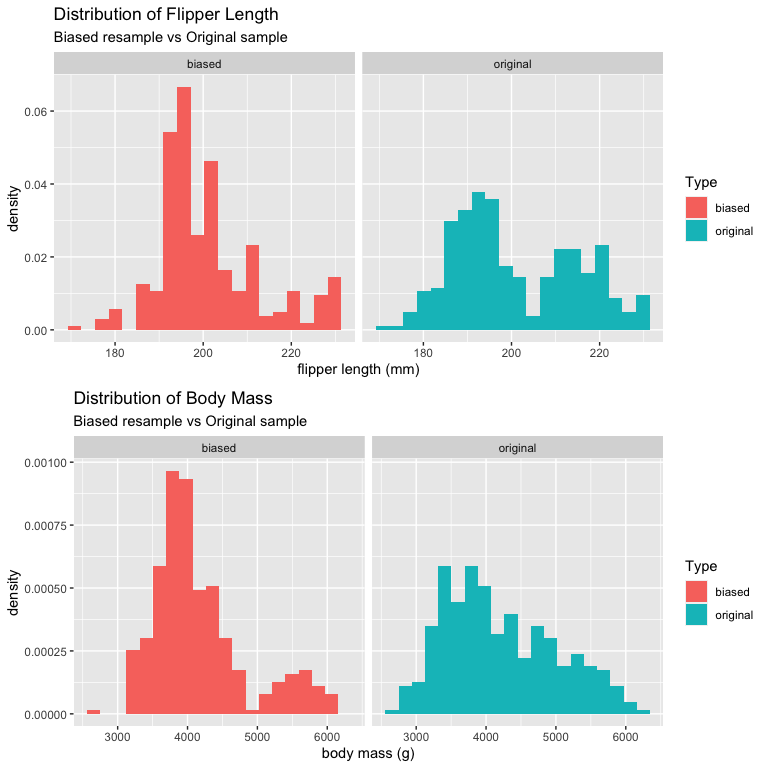The result shows that a bias is induced, but as a result, variance for all dimensions decrease as there are less unique points sampled. The volume will also be significantly reduced if the applied bias is strong. Therefore, it is recommended to only apply strong bias to larger datasets. In this example, sigma is chosen arbitrarily as twice the variance of the original columns. The larger sigma is, the weaker the bias and vice versa.

## Use case 3: Using overlap statistics to test for similarity of populations

The following code demonstrates how to test the null hypothesis that two samples come from the same distribution. In this example, we map the longitude and latitude data from quercus to a four dimensional climate space, as in demo(quercus).

To test whether the two species Quercus rubra and Quercus alba have the same climate niche, there are two approaches. In the first approach, we use the combined sample data as an approximation of the true distribution. To generate the null distribution for overlap statistics, we treat all of the data as having the same label and then bootstrap hypervolumes from the combined data. The overlaps of the resampled hypervolumes are used to generate the distribution of the overlap statistics. If the size of the two samples is the same, the function takes half the hypervolumes and overlaps them with each of the other hypervolumes. In this case, since the number of samples of Quercus rubra and Quercus alba are different, we need to bootstrap an equal number of hypervolumes for each sample size.

The second approach is a permutation test. For this method, the labels of the data are rearranged then the data is split by label. A pair of hypervolumes are generated from each split and overlap statistics are generated from each pair.

The benefit of the first method is the ability to generate multiple overlap statistics per hypervolume. If both methods generate $$N$$ hypervolumes, the first method will generate $$\frac{N^2}{4}$$ overlap statistics while the second method will generate $$\frac{N}{2}$$ overlap statistics. Since hypervolume construction and overlap both can be non-deterministic processes, method one will account for more of the variance from generating the overlap. However, when sample size is small, the combined data may not be a good approximation of the population. In this case, it is better to use method two, because it does not make any assumptions about the population, and generating more hypervolumes is fast for smaller sample sizes.

data("quercus")
data_alba = subset(quercus, Species=="Quercus alba")[,c("Longitude","Latitude")]
data_rubra = subset(quercus, Species=="Quercus rubra")[,c("Longitude","Latitude")]
climatelayers <- getData('worldclim', var='bio', res=10, path=tempdir())

# z-transform climate layers to make axes comparable
climatelayers_ss = climatelayers[[c(1,4,12,15)]]
for (i in 1:nlayers(climatelayers_ss))
{
climatelayers_ss[[i]] <- (climatelayers_ss[[i]] - cellStats(climatelayers_ss[[i]], 'mean')) / cellStats(climatelayers_ss[[i]], 'sd')
}
climatelayers_ss_cropped = crop(climatelayers_ss, extent(-150,-50,15,60))

# extract transformed climate values
climate_alba = extract(climatelayers_ss_cropped, data_alba)
climate_rubra = extract(climatelayers_ss_cropped, data_rubra)

# Generate Hypervolumes
hv_alba = hypervolume(climate_alba,name='alba',samples.per.point=10)
hv_rubra = hypervolume(climate_rubra,name='rubra',samples.per.point=10)

# Method 1: 2hr runtime with 12 threads
combined_sample = rbind(climate_alba, climate_rubra)
population_hat = hypervolume(combined_sample)

# Create bootstrapped hypervolumes of both sample sizes
method1_path_size_1669 = hypervolume_resample("quercus_1669_boot", population_hat, "bootstrap", n = 100, cores = 12)
method1_path_size_2110 = hypervolume_resample("quercus_2110_boot", population_hat, "bootstrap", n = 100, cores = 12)

result1 = hypervolume_overlap_test(hv_alba, hv_rubra, c(method1_path_size_1669, method1_path_size_2110), cores = 12)

#Method 2: 9hr runtime with 12 threads
method2_path = hypervolume_permute("rubra_alba_permutation", hv1, hv2, n = 1357, cores = 12)

result2 = hypervolume_overlap_test(hv1, hv2, method2_path, cores = 2)

# Graphical Results of null sorensen statistic
plot1 = result1$plots$sorensen + ggtitle("Method 1", as.character(result1$p_values$sorensen)) + xlab("Sorensen Index")
plot2 = result2$plots$sorensen + ggtitle("Method 2", as.character(result2$p_values$sorensen)) + xlab("Sorensen Index")
grid.arrange(plot1, plot2, ncol=2)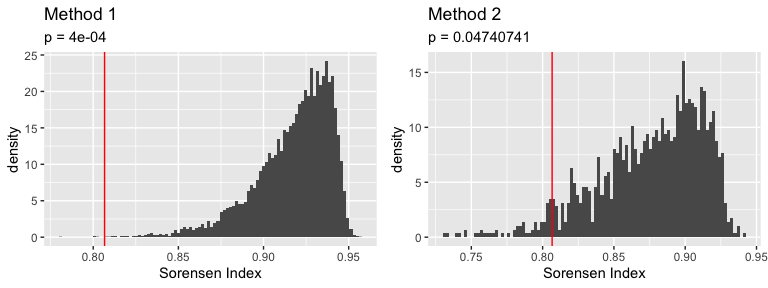For our example, the red line shows the observed value of the Sorensen overlap index. Method one results in a significantly lower p value, but Method two also results in a low p value. Since p is less than 0.05 in both cases, we can reject the hypothesis that the two Quercus species have identical climate niches.